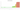# ج.م.‏

## Egyptian Pound

Egypt's official currency is the pound. It is split up into 100 piastres, 1,000 milliemes, or ersh.

#### how has the value of the currency changed in the last year?## USD 1 = EGP 30.895

How much is10 US dollars worth inEgyptian pounds?
At the current exchange rate, 10 US dollars is worth 308.95 Egyptian pounds
How much is50 US dollars worth inEgyptian pounds?
At the current exchange rate, 50 US dollars is worth 1,544.75 Egyptian pounds
How much is100 US dollars worth inEgyptian pounds?
At the current exchange rate, 100 US dollars is worth 3,089.5 Egyptian pounds
How much is500 US dollars worth inEgyptian pounds?
At the current exchange rate, 500 US dollars is worth 15,447.5 Egyptian pounds
How much is2,000 US dollars worth inEgyptian pounds?
At the current exchange rate, 2,000 US dollars is worth 61,790 Egyptian pounds
How much is10 US dollars worth inEgyptian pounds?
At the current exchange rate, 10 US dollars is worth 308.95 Egyptian pounds
How much is50 US dollars worth inEgyptian pounds?
At the current exchange rate, 50 US dollars is worth 1,544.75 Egyptian pounds
How much is100 US dollars worth inEgyptian pounds?
At the current exchange rate, 100 US dollars is worth 3,089.5 Egyptian pounds
How much is500 US dollars worth inEgyptian pounds?
At the current exchange rate, 500 US dollars is worth 15,447.5 Egyptian pounds
How much is2,000 US dollars worth inEgyptian pounds?
At the current exchange rate, 2,000 US dollars is worth 61,790 Egyptian pounds